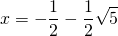# Solution assignment 08 The basics

### Assignment 8

Solve the following equations: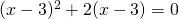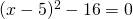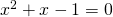### Solution

We can solve the first equation by taking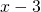outside brackets. We get: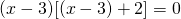, so: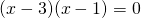, dus: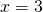of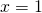We can write the second equation as: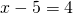of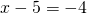, so: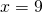ofThe last equation cannot be solved by factorizion. Then the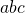-formula has to be used and always delivers a solution if it exists. We get: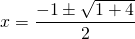, and thus: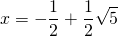of